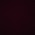## 28 July 2015

### Answer key with explanations to CEED 2015 previous paper

-------------------------------------------------------------------------------------------

This post serves as an answer key to CEED 2015 official test. I tried my best to give detailed explanations for most of the questions your benefit.

You can get the downloadable question paper here

### Solutions :

1)

The situation is given in the below picture. H stands for home, M for market, s for school, PO for post office.

It's given in the question that when Alakananda travels exactly north from Market, she reaches Home, and it's also given that walking from school exactly in east 37km, will end up at Market. and from market, if she move towards east, she will reach to Post office. So, School, Market and Post office should lie on the same straight line.

According to the triangle formed by SHM, applying Pythagoras theorem
x = sqrt(37^2 - 35^2)

You don't even need calculator to solve this, just apply (a^2 - b^2) formula, which is equal to (a+b)(a-b)
so,
x = sqrt((37+35)(37-35))
= sqrt(72*2) = sqrt(144) = 12

Now, according to the triangle formed by H-M-PO, applying Pythagoras theorem
y = sqrt(13^2 - x^2)
y = sqrt(13^2 - 12^2) = 5
So, distance from school to Post office = 35+y = 40

2)

The below shown picture illustrates the situation for 6X6X6 cube. Cubes which are in edges (other than the corners) will have red color on their two faces.
Consider the front face, if we were to cut the cube into small cubes of 1X1X1 shapes, then we need to make cuts as indicated by the straight lines (other than the edges) shown in the above explanatory image. For the given cube of size 6*6*6, we need to make 5*5*5. If we do so, then the individual cubes that are marked with symbols (as shown in the above examples for front side and side1) will have two surfaces with red.

So, counting the total number of cubes on corners/edges (which is 8+8+6+6 = 28 for a single side - for the present case of 8*8*8 cube) and subtracting 8 (removing 8 corners which has red color on three surfaces) will give the answer
i.e
(28+28+12+12)-8 = 72 cubes

3)

The given solid is symmetry but it has slant shapes, so should be careful while counting.
Try this way -

Avoid counting the slant surfaces (rectangles in the given picture) and count the rest shapes as I have given (front-right-back-R-Top-bottom).
After this, count the slant surfaces separately.

From front (excluding slanting rectangles) -
1 small square + four triangles = 5 surfaces

The picture is symmetry, so from all sides, the same count will come, i.e
1 small square + four triangles = 5 surfaces

So, since we have to count fro six sides, so total surfaces (excluding slant rectangles) = 5*6 = 30

Now, separately count the slant rectangles - which will be 12 (be careful while counting this). So, total = 30+12 = 42

You may check the above image to get a clear understanding of the explanation.

4)

Below explanation will help you solve this questions

5)

This question is also based on observation. Since the geometry is symmetry, we can take a quarter of the symmetrical shape, and count the number of cubes, and multiply that by 4. Be careful while counting the central cubes. Better if you count them separately!
Counting this way, Answer = 11*4 + 15 = 59

6)

M (all) ---> F (all) ---> D
M (all) ----------------> D

So, all Mammals are dolphins
Also, since all mammals are fish, obviously part of the fish are mammals, so Option A is also correct, B is wrong, straight forward. C is also logically correct.

7)

Check the shades of Red color - wiki page
Learn about shades of other colors - wiki page

8)

That's why it has no/least bonnet at the front!

9)

I guess it's M, e, s
But in answer key it's mentioned as a,c

10)

All the films are Steven Spielberg hit movies

11)

check the complete collection of Pablo Picasso works
A very good facts blog about famous persons around the world
Also for reference - 100 greatest paintings of all time

12)

This question is also based on your observation, anyway here's a simple procedure or way of observing the image.
In the given picture, assume two arrows diagonally (indicated in red). Now; note the shapes at the corners - for example one is semi rectangle. and the shape in opposite side is say triangle. Now only consider the yellow arrows, which are exactly opposite. Note the shapes - semi circle and gun shape, now observe all the options which have this pattern.
Also, don't forget the shape of the center black rectangle as per the rotated orientation of the options.

13)

Get the complete list with more details about Font - wiki page

14)

Check here - Photography guide for Part-A

15)

16)

As shown in the figure, consider the first image with black face on the top and red face on the front. Now, we want the color of the surface on the opposite side of the black surface. So, tilt/turn the cube in such a way that black surface is moved to the back position as shown in the next image of the below picture. I mean turn the cube clockwise. Now, red face is on the top, while green surface is still on the right side of the cube view. Now check the options with this configuration, only last picture in the question matches. So, it's yellow color !

If you were asked to find different color - other than yellow, do the above procedure and orient the cube such that it matches color arrangements as in any one of the option given to you.

17)

Split the combinatorial words into two parts as shown below
wigges + slorm -----------> flat + pen
widdle + brap -------------> round + nib
slorm + wiggel ------------> pen + ink

from this we can conclude that
wigges ---> flat
slorm -----> pen
brap ------> nib
wiggel -----> ink

now we want to find 'nibstore' = nib + store = brap + ........(something)
option a) brap+dansa
option d) brap + wiggel ( which is brap+ink ....... incorrect)
so option a should be the answer.

18)

This problem can be solved using your imagination/observation. Anyway as usual let me give one possible method

Method
Figure shows one possible shape of the nib which can draw as shown in the question. Remember Indians have the habit of drawing from left to right. And the given straight line is projected towards left. So, the nib should also project towards left as I showed in the picture.
Now, if you are to draw a circle, you will obviously start from the top and move in anti clockwise direction as shown in the image (b). Now, if you use the given nib, ans start drawing a circle, then you have to expect a sharp line (instead of broad width line) at the place where the nib moves parallely in the direction of the nib angle. To make it simple, if you assume tangent lines, drawn over the circle (b) as shown in (c)then the location on the circle at which the tangent angle is same as the nib angle is where the line will not be thick, got it ?
So, using this logic, option C is the answer.

19)

Here's - a good guide for art movements
Go through this wiki page in free time

20)

learn different color combinations. There's also a detailed discussion regarding CMYK and RGB color modes in that link. You may find that useful.

21)

Here goes the explanations
Kharavela, was the third and greatest emperor of the Mahameghavahana dynasty of Kalinga (present-day Odisha). The main source of information about Kharavela is his famous seventeen line rock-cut Hatigumpha inscription in a cave in the Udayagiri hills near Bhubaneswar, Odisha (source : wikipedia)

Learn about - Indian epigraphy history
Learn about - Famous inscription in India

22)

It's straight and I guess I don't have to explain this. If you still have doubts, then you may drop a comment, I will give proper explanations like I did for other questions.

23)

check - materials and manufacturing process

24)

Check here - List of Indian Folk dances (state-wise)
A complete list of - Indian Folk dance (wiki page)

25)

Go through this link to learn about famous books and others

26)

Check - Logos collection of Indian organizations

27)

28)

I prepared the below picture by marking possible similar lines in the given options using distinguishable colors. As you can visualize, option b is the correct one, while in other options, pattern varies in one way or other.

29)

check - Working on reflections and geometrical solids

30)

The below picture helps in better understanding, although I used lines (with diff. colors) to extend and show where they meet. Clearly, red extended lines are out of perspective while others are able to meet at a vanishing point. Although I used coloured lines for your explanation, but you need to imagine and visualize this while in exam.

31)

I initially got confused a bit with this question. They mentioned that the arm has to be turned toward us. If we assume that that arm is of some one else in the screen and has to be turned toward us (which means outside of the screen and towards us), then option A is not the answer. But according to the answer key and Option A being the answer, we can say that the image shown has to be assumed as our own arm, and moving toward us means moving away from the screen. In this case option A is the answer.

The reason is when the arm moves away from us, then hands with fingers will look smaller in size compared to the arm/upper limb since they are near to the scene of capture. Things that are near to the camera should appear bigger in size compared to farther objects. Also notice the fold shown near elbow.

32)

No matter what distance the person moves, his height will be same, to find the mid point, use the concept of maths! That is the point of diagonal is always at the mid, like for the example of a diagonal shown below

So, if you draw two diagonal lines, then the contact point is obviously the mid path! as shown in the figure

33)

If you observe the middle shape (stick human figure), then he is not at the maximum height nor is falling down (In terms of physics - he is in the travel of ascent). In other words, he is moving away from earth (against the gravity) ans so the air will try to push hum down. It also tries to push his cape to the down. So, it's almost vertically downward - Option A matches.

If he were to jump from top, or he is falling down, then the air will again try to oppose him in opposite direction to his travel, In other words towards up. So, the direction of the cape should also be vertical (In actual, the direction would most probably be in the curve (parabola) of the travel/path.

34)

Figure clearly explains the situation. Like in the above questions, use the principle of numbering system. Now, identify the projections and mark them as I did in the picture - Porjecion 1, proj 2, proj 3
Now, check the options that have depressions at the same location as projections are in the given question. Option C matches as shown in the picture.

35)

You can learn more about Geometry transformations in Part A Resources page

36)

The below picture helps you with explanation.

As can be seen, if the top portion is circular (just like a pipe), then the front view will be as shown in the left most image above. Both the cone circular section and the pipe circular section matches horizontally and so appears like a line in front view. But if instead the pipe is rectangular in shape, then as shown in the third (right most) image above, the extra projections (denoted by A,B,C,D) are over-fit at the intersection ad their radius is greater compared to the radius of a circle inscribed inside the square/rectangle. So, it projects and hence extends downwards in the top view with sharp edges as shown in option A. Hope you got it!

(Check the above links for similar geometry study)

37)

(By observation)

38)

It can be solved by visual observation without any hand work. If you are not able to do so, then follow the following method

Method :
Give numbering to the three rectangular projections of the given image. Three projections are there, so three numbers, follow either clockwise or anticlockwise numbering system.
Start from a reference side, say right side of the given question. Remember this reference. Now, take all the options (one by one) and try to follow the same numbering system in the same direction as done to the question. Identify where the reference side is and start from there. Visually check the distance between the number sequence in the assumed direction. If it's in correct order, then list those options.

Now check the size of the projections at each numbered mark. If it matches at all numbers, then that would be the answer. Only last option is matching

39)

In thermal engineering terms, that's a combination of conduction and convection heat transfer process. Heat from outside as well as from inside will be taken by the wet sand (between the two pots) and since sand has greater pores and is not compact, there will be minute spaces through which air can easily flow. This air will come in contact with the wet sand and will take away the heat by evaporating. Thus the water inside will not be affected by heat outside, which is one best way of natural cooling that is practiced in India too !

40)

41)

'Design of everyday things' is a popular book in the world of design and is written by Donald Norman. You can get the downloadable form of this book in this blogs resource page.

42)

(By observation)
You have to proceed in reverse direction, like they have mentioned fold 1 and fold 2 right, now considering the image 1 (folded sheet with cut), remove the fold as in fold 2 position, then unwrap the sheet as in fold 1 position.

43)

Brahmagupta was an Indian mathematician and astronomer. Brahmagupta was the first to give rules to compute with zero.

44)

45)

46)

47)

Remaining font styles have narrower body. Cambria comes under serif category while the rest are sans-serif (source : wiki)

48)

As indicated by arrows in the below picture, consider the X tag, marked by the blue lines. On the x side, two arrows are punched and the direction of the arrows (two numbers) point towards x mark. Note this arrangement. Now as pointed by the red mark, on y side, only one arrow is punched such that it is pointing away from the Y mark. got it ? I have also sketched this separately as shown in the picture.

Check the option which exhibits this behaviour/pattern. Option b is the only one matching. We then don't have to bother about any other punches as given additionally in the options

49)

Answer : b (as in CEED official Key)
Frankly speaking I'm not actually good at Fonts. Please check yourself.

50)

Already there are 29 states (including UP - as a single state) and when UP gets divided into 4 states, we need to add three (since already one state is considered earlier). So, now total = 29+3 = 32 states

#### Also see

Like Stuff You Look blog on Facebook page to get updated with latest posts.

1.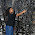i have doubt in question 15..https://www.youtube.com/watch?v=5IlrOxRPy0U as per this video tetra packs are made of paper also which is not mentioned in the answer

1.That was doubtful to me when I was preparing these answers, that's y didn't marked option 'a'. I will first confirm myself and update accordingly. Thank you for pointing out this to me.

2.Hi Bhanu can u provide me explanation for Q 32. I know its observation based but i am not able to get it.

3.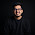Can you give me solution for Q36 PLEASE _/\_

1.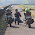The base solid is a cone. When you cut a cone from below in this case a square, semi circular projections would remain because of the slant nature of the solid. Hence, the answer is A.

4.Upload solution for CEED 2015 Part - B.
Thanks for uploading solutions for previous years

5.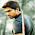ans of Que 37 is B)

6.C is correct ans if we r placing sixth block on upper side

7.I think the answer of que no4 is 93...the 1st number is 12 then 2nd is 24 then the 3rd is the swappwed version of 1st number 12 i,e 21 4th numder is the swapped version of 24 i.e 42 so the swapped version of 39 is 93

1.what if there is some number like 50, how will u swap and mention it. and are u not satisfied with the mathematical explanation that I gave above ?

2.Yes i am satisfied no doubt its correct,but d way i thought somebody else may also think that way..because the logic i gave is the immediate thought which will come in mind.wont be it correct...and plz help me with que no 11 ootion c..is it mf hussain???

3.12(x2) 24

(24-3)21(x2) 42,

(42-3) 39(x2) 78

(78-3) 75 (x2) 10

4.That's the beauty of maths,
most problems can be solved in multiple way, your way of answering is also correct. Thanks!

8.Q11 option C????

1.C is not made by Picasso as per my knowledge, if u have come across any web page or source stating that the art was done by picasso, pl share here, i will correct the options

9.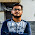Hello Bhanu sir, am not getting ans of 32,36,37. can u please help..i know its all about self observation..bt still am not coming to the point!!!!!

1.I explained q32 above in the ques, u can chk now. Q36, 37 is not that easy to explain in written unless u r sitting nxt to me :P

10.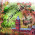hello sir thank you so much for your great help to us. whatever u proceed in top of this page everything is extraordinary except which i have doubt an a question. can i say that?

1.11.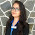ans. of ques 4 my way...
12*2=24
21*2=42
39*2=78...24-3=21, 42-3=39, so ths way 78-3=75....my way liitl wierd but i got the answer... :)

1.Ya, I know, most math problems can be solved in many ways.I already mentioned this in above comments.

12.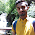sir plzz cud u explain q.36 y can't it be C??

1.Explanation with an image is updated now. You can check the answer once again to clarify yourself.

13.o.k. thanks a lot sir..

14.Sir You Have A Great Blog!!!
Its just Awesome
You Mentioned an answer key in solution of Q9, where can I find it?
Otherwise could you Upload it?

1.2.Thanks
And do you also have the CEED 2016 Paper?
I am unable to find it online even though it has happened.

3.CEED'16 questions paper will not be released, at least in this week. It takes time. Better don't wait for that if u r planning for this years UCEED!

15.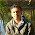12*2=24
24-(1+2=3)=21
21*2=42
42-(2+1=3)=39
39*2=78
78-(3+9=12)=66
so answer is 66 is this logic right

1.Which ques ?

16.2015 part B solution?

17.Hi Bhanu Please upload CEED 2015 and 2016 part B solutions.. Thanks in advance :)

18.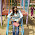please explain question 22. Thank you

19.Hi, this is really very helpful.
But can you please explain me the 36th question?

20.I think B option should be the answer of question 37 as in the image, first block corners are touching the sides of the block beneath it and option B shows the same figure if someone watches it from top which clearly signifies that that the B option is correct not the C option.

1.They are following a particular sequence that is the extruded part of 3rd block is little less than that of 2nd, extruded part of 4th is less than that of 3rd and 5th block is exactly touching the edges of 4th block hence the 6th box has to be inside 5th block. They are asking about 6th block read the question properly

21.This comment has been removed by the author.

1.figure is shown for 6*6*6 but I had given explanation for 8*8*8 only. I think u haven't gone thru the explanation for that answer

22.in q5 you forgot to count the big cube formed by smaller one in center

23.Question 37.... answer (C) is wrong, right answer is (b). in given question, top square is touching the boundaries of the 2nd square (from top) and in answer (c) top square in untouched..,, pls explain...

1.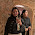Same query. please exlain.

2.read the ques carefully it's given when you add a 6th block to it

24.hello sir,
can you please post the answers and explanations to UCEED 2017 paper and any guidelines for 20th January.

1.chk that in this pg
25.